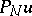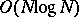# Fourier pseudo-spectral method

(diff) ← Older revision | Latest revision (diff) | Newer revision → (diff)

A type of trigonometric pseudo-spectral method (cf. Trigonometric pseudo-spectral methods) used to solve differential and integral equations. See also Chebyshev pseudo-spectral method.

The Fourier pseudo-spectral method is used for problems in which there is a natural periodicity. In multi-dimensional problems it should be used only in those directions with periodic boundary conditions. In non-periodic directions, Chebyshev methods, finite element or difference methods of high order should be used.

Suppose the equation $L u = f$ is to be solved where $L$ is a differential operator, $f$ is a given periodic function and $u$ is an unknown periodic function (period $2 \pi$). In the Fourier pseudo-spectral method, the solution $u$ is approximated by a trigonometric polynomialthat interpolates $u$ at equally spaced points $x _ { j } = \pi j / N$, $j = 0 , \ldots , 2 N - 1$.

The Lagrange interpolation polynomial (cf. also Lagrange interpolation formula) has the form $P _ { N } u = \sum _ { j = 0 } ^ { 2 N - 1 } u ( x _ { j } ) C _ { j } ( x )$, where $C_{j}$ is the Cardinal function, having the form

\begin{equation*} \frac { 1 } { 2 N } \operatorname { sin } N ( x - x _ { j } ) \operatorname { cot } \frac { ( x - x _ { j } ) } { 2 }. \end{equation*}

An equivalent way of defining the interpolating polynomial is by the discrete Fourier transform: $P _ { N } u = \sum _ { k = - N } ^ { N } a _ { k } e ^ { i k x }$, where the Fourier coefficients are

\begin{equation*} a _ { k } = \frac { 1 } { 2 N c _ { k } } \sum _ { j = 0 } ^ { 2 N - 1 } u ( x _ { j } ) e ^ { - i k x _ { j } }, \end{equation*}

\begin{equation*} c _ { N } = c _ { - N } = 1 , c _ { j } = 2 \text{ otherwise}. \end{equation*}

In the Lagrange polynomial or "grid-point representation" , the problem can be written as $L _ { i , j } u _ { j } = f _ { i }$, where $L _ { i ,\, j } = L C _ { j } ( x ) |_ { x = x _ { i } }$ The form of $L_{i ,\, j}$ can be found through differentiation of the Cardinal function: $C _ { j } ( x _ { i } ) = \delta _ { i , j }$,

\begin{equation*} \frac { d C _ { j } } { d x } ( x _ { i } ) = \left\{ \begin{array} { l l } { 0 } & { \text { for } i = j, } \\ { \frac { 1 } { 2 } ( - 1 ) ^ { i + j } \operatorname { cot } \frac { x _ { i } - x _ { j } } { 2 } } & { \text { for } i \neq j, } \end{array} \right. \end{equation*}

\begin{equation*} \frac { d ^ { 2 } C _ { j } } { d x ^ { 2 } } ( x _ { i } ) = \left\{ \begin{array} { l l } { - \frac { 2 N ^ { 2 } + 1 } { 6 } } & { \text { for } i = j, } \\ { \frac { 1 } { 2 } \frac { ( - 1 ) ^ { i + j + 1 } } { \operatorname { sin } ^ { 2 } \frac { x _ { i } - x _ { j } } { 2 } } } & { \text { for } i \neq j, } \end{array} \right. \end{equation*}

to give a few. The derivative matrices are full and $O ( N ^ { 2 } )$ operations are required for evaluation.

The problem can also be formulated using the spectral coefficient representation. Derivatives are found through differentiation of the discrete Fourier series, for example,

\begin{equation*} \left( \frac { d } { d x } \right) ^ { 2 } P _ { N } u ( x ) = \sum _ { k } ( i k ) ^ { 2 } a _ { k } e _ { i k x }, \end{equation*}

and have an $O ( N )$ operation count. If the operator $L$ is non-linear or has non-constant coefficients multiplying the derivatives, evaluation of $L$ is much more complicated.

The fast Fourier transform provides a way of switching between grid-point and spectral representations inoperations, instead of the $O ( N ^ { 2 } )$ using the definitions above. Derivatives can be accomplished in spectral space and multiplication of non-constant coefficients or evaluation of non-linear terms can be accomplished in grid point space — each with $O ( N )$ operations.

Particularly for time-dependent problems in which boundary value problems must be solved at each time step, it is very important to minimize operation counts by taking full advantage of each representation and the fast transformation between representations.

As an example, consider the operator

\begin{equation*} L u = \operatorname { sin } ( x ) \frac { d ^ { 2 } u } { d x ^ { 2 } } - ( \frac { d u } { d x } ) ^ { 2 } \end{equation*}

for the time-dependent problem $d u / d t = L u$. Suppose at a particular time the spectral coefficients $a _ { j }$, $j = 0 , \dots , N - 1$, are known. To compute $Lu$, first the spectral coefficients of the first- and second-order derivatives are found:and $- j ^ { 2 } a_j$. Then both of these arrays are transformed into their grid point representation using a fast Fourier transformation. Then $Lu$ can be evaluated in $O ( N )$ operations. The complete evaluation of the operator is done inoperations rather than $O ( N ^ { 2 } )$ if done completely in one space. This property becomes even more critical for multi-dimensional problems; the operation counts are $O ( N ^ { d } \operatorname { log } N )$ versus $O ( N ^ { 2 d } )$ for a $d$-dimensional problem.

How to Cite This Entry:
Fourier pseudo-spectral method. Encyclopedia of Mathematics. URL: http://encyclopediaofmath.org/index.php?title=Fourier_pseudo-spectral_method&oldid=50662
This article was adapted from an original article by Richard B. Pelz (originator), which appeared in Encyclopedia of Mathematics - ISBN 1402006098. See original article